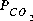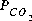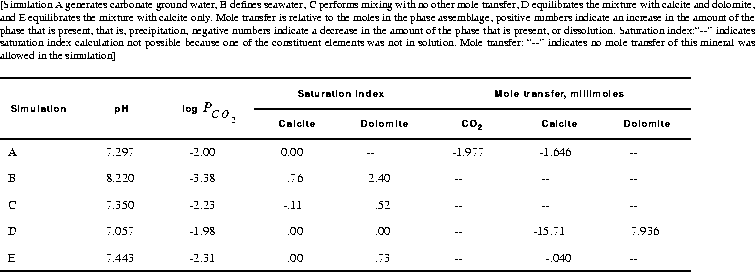[Next] [Previous] [Up] [Top]

EXAMPLES

## Example 3.-- Mixing

This example demonstrates the capabilities of PHREEQC to perform a series of geochemical simulations, with the final simulations relying on results from previous simulations within the same run. The example investigates diagenetic reactions that may occur in zones where seawater mixes with carbonate ground water. The example is divided into five simulations, labeled A through E in table 7. (A) Carbonate ground water is defined by equilibrating pure water with calcite at aof 10-2.0 atm. (B) Seawater is defined using the major-ion data given in table 2. (C) The two solutions are mixed together in the proportions 30 percent seawater and 70 percent ground water. (D) The mixture is equilibrated with calcite and dolomite. Finally, (E) the mixture is equilibrated with calcite only to simulate slow reaction kinetics of dolomite.

### Table 7. Input data for example 3

```TITLE Example 3, part A--Calcite equilibrium at log Pco2 = -2.0 and 25C.
SOLUTION 1  Pure water
pH      7.0
temp    25.0
EQUILIBRIUM_PHASES
CO2(g)          -2.0
Calcite         0.0
SAVE solution 1
END
TITLE Example 3, part B--Definition of seawater.
SOLUTION 2  Seawater
units   ppm
pH      8.22
pe      8.451
density 1.023
temp    25.0
Ca              412.3
Mg              1291.8
Na              10768.0
K               399.1
Si              4.28
Cl              19353.0
Alkalinity      141.682 as HCO3
S(6)            2712.0
END
TITLE Example 3, part C--Mix 70% ground water, 30% seawater.
MIX 1
1      0.7
2      0.3
SAVE solution   3
END
TITLE Example 3, part D--Equilibrate mixture with calcite and dolomite.
EQUILIBRIUM_PHASES 1
Calcite         0.0
Dolomite        0.0
USE solution 3
END
TITLE Example 3, part E--Equilibrate mixture with calcite only.
EQUILIBRIUM_PHASES 2
Calcite         0.0
USE solution 3
END
```
The input for part A (table 7) consists of the definition of pure water with SOLUTION input, and the definition of a pure-phase assemblage with EQUILIBRIUM_PHASES input. In the definition of the phases, only a saturation index was given for each phase. Because it was not entered, the amount of each phase defaults to 10.0 mol, which is essentially an unlimited supply for most phases. The reaction is implicitly defined to be the equilibration of the first solution defined in this simulation with the first pure-phase assemblage defined in the simulation. (Explicit definition of reaction entities is done with the USE keyword.) The SAVE keyword instructs the program to save the solution following the final (and only in this example) reaction step as solution number 1. Thus, when the simulation begins, solution number 1 is pure water. After the reaction calculations for the simulation are completed, the composition of the water that is in equilibrium with calcite and CO2 replaces pure water as solution 1.

Part B defines the composition of seawater, which is stored as solution number 2. Part C mixes ground water, solution 1, with seawater, solution 2, in a closed system in whichis calculated, not specified. The MIX keyword is used to define the solutions and mixing fractions. The SAVE keyword causes the mixture to be saved as solution number 3. The MIX keyword allows the mixing of an unlimited number of solutions in whatever fractions are specified. The fractions need not sum to 1.0. If the fractions were 7.0 and 3.0 instead of 0.7 and 0.3, the mass of water in the mixture would be approximately 10 kg instead of approximately 1 kg, but the concentrations in the mixture would be the same as in this example. However, during subsequent reactions it would take approximately 10 times more mole transfer to equilibrate with the phases, that is, to produce the same concentrations as in this example.

Part D equilibrates the mixture with calcite and dolomite. The USE keyword specifies that solution number 3, which is the mixture from part C, is to be the solution with which the phases will equilibrate. By defining the phase assemblage with "EQUILIBRIUM_PHASES 1", the phase assemblage replaces the previous assemblage number 1 that was defined in part A. Part E performs a similar calculation to part D, but uses phase assemblage 2, which does not contain dolomite as a reactant.

### Table 8. Selected results for example 3Selected results from the output for example 3 are presented in table 8. The ground water produced by part A is in equilibrium with calcite and has a logof -2.0, as specified by the input. The moles of CO2 in the phase assemblage decreased by about 2.0 mmol, which means that about 2.0 mmol dissolved into solution. Likewise, about 1.6 mmol of calcite dissolved. Part B defined seawater, which is calculated to have slightly greater than atmospheric carbon dioxide (-3.38 compared to about -3.5), and is supersaturated with calcite (saturation index 0.76) and dolomite (2.40). No mole transfer was allowed for part B. Part C performed the mixing with no additional reactions. The resulting logis -2.23, calcite is undersaturated and dolomite is supersaturated. The saturation indices indicate that thermodynamically, dolomitization should occur, that is calcite should dissolve and dolomite should precipitate. Part D calculates the amounts of calcite and dolomite that should react. To produce equilibrium 15.7 mmol of calcite should dissolve and 7.9 mmol of dolomite should precipitate. Dolomitization is not observed to occur in present-day mixing zone environments, even though dolomite is the thermodynamically stable phase. The lack of significant dolomitization is due to the slow reaction kinetics of dolomite formation. Therefore, part E simulates what would happen if dolomite does not precipitate. If dolomite does not precipitate, only a very small amount of calcite dissolves (0.04 mmol) for this mixing ratio.

Table 7. Input data for example 3
Table 8. Selected results for example 3

User's Guide to PHREEQC - 07 MAY 96
[Next] [Previous] [Up] [Top]